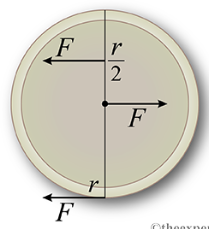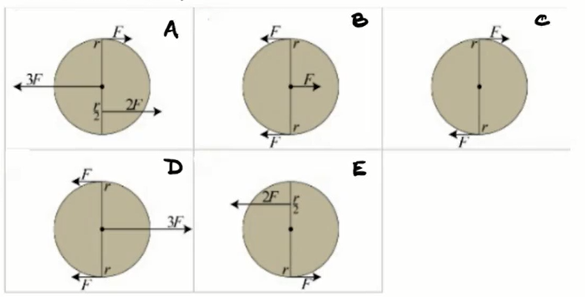Problem: A circular air hockey puck of radius r slides across a frictionless air hockey table and is subjected to several forces as shown below. The magnitude and direction of each force is given. Forces are applied at either the center of mass of the puck, the outer edge (a distance r from the center), or a distance halfway (r/2) between the center and the outer edge. Select the examples below that have a net torque of zero about the axis perpendicular to the page and extending from the center of the puck.

82% (94 ratings)

Torque, τ,

82% (94 ratings)
Problem Details

A circular air hockey puck of radius r slides across a frictionless air hockey table and is subjected to several forces as shown below. The magnitude and direction of each force is given. Forces are applied at either the center of mass of the puck, the outer edge (a distance r from the center), or a distance halfway (r/2) between the center and the outer edge.Select the examples below that have a net torque of zero about the axis perpendicular to the page and extending from the center of the puck.